# Magnetic field inside a solenoid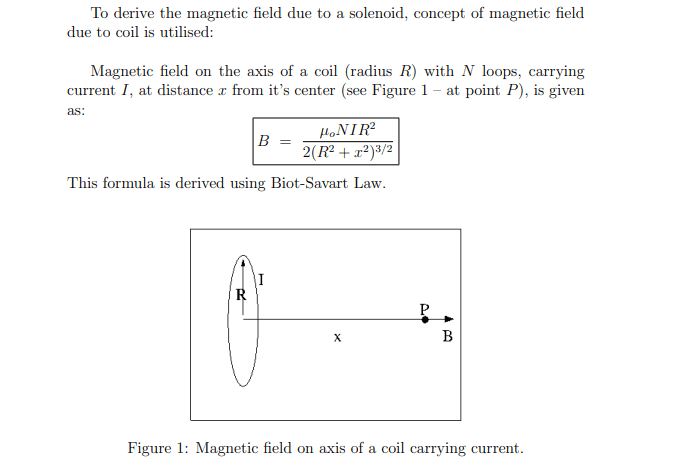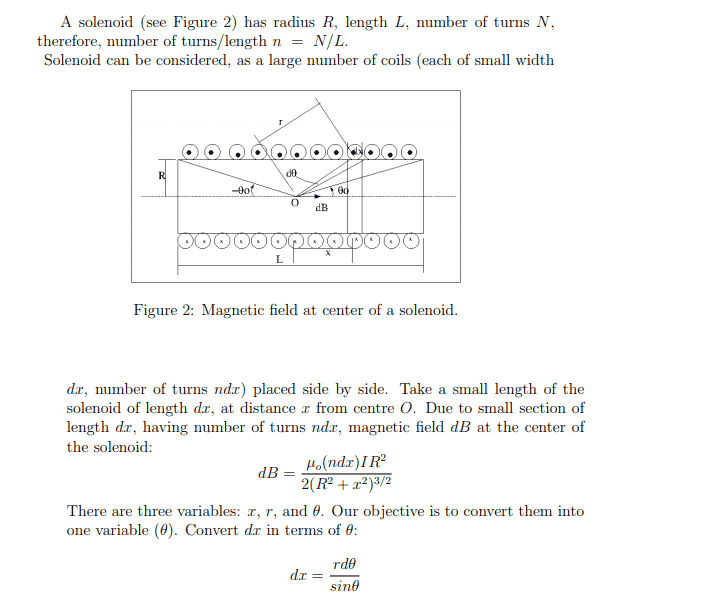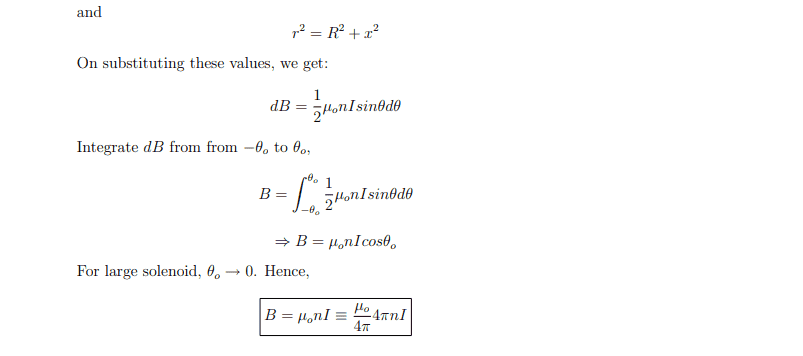I have a problem with the derivation above I dont get how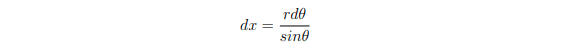Can someone derive this and illustrate this visually for example by using Figure 2 or using another drawing?

PeroK
Homework Helper
Gold Member
2020 Award
It looks wrong to me. The book should have ##\theta## going clockwise with ##\theta = 0## as the vertical, and be integrating from ##-\frac{\pi}{2} + \theta_0## to ##\frac{\pi}{2} - \theta_0##.

It looks like the book has two wrongs making a right.

PS with the above I get ##dx = \frac{r d\theta}{\cos \theta}##

well it is not from a textbook it is a document I recieved. Unfortunately I am a bit lost from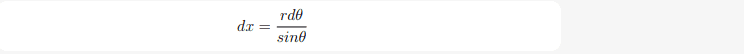and the equations afterwards. Could you derive how to get to: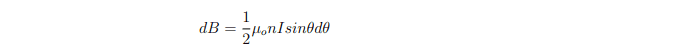starting from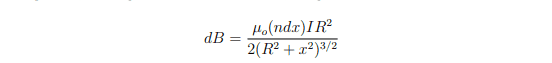PeroK
Homework Helper
Gold Member
2020 Award
well it is not from a textbook it is a document I recieved. Unfortunately I am a bit lost from
View attachment 254268

You're lost because that is wrong. Try what I posted.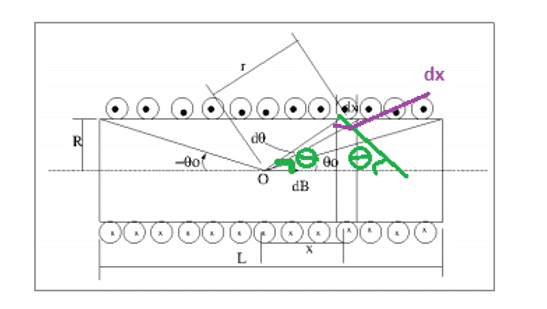I have tried to illustrate my problem in the drawing above. Since ##r d\theta## is normal to the radius r and approximately linear since it is a short part of the bowlength the angle between dx and ##r d\theta## should be the same as the angle between r and x that makes cosinus. But what if the fraction ##\frac{r d\theta}{dx}## and the ##\frac{r }{x}## is not the same? We don't know the length of ##r d\theta## measured up to r and the length of dx measured up to x?

256bits
Gold Member
View attachment 254289

I have tried to illustrate my problem in the drawing above. Since ##r d\theta## is normal to the radius r and approximately linear since it is a short part of the bowlength the angle between dx and ##r d\theta## should be the same as the angle between r and x that makes cosinus. But what if the fraction ##\frac{r d\theta}{dx}## and the ##\frac{r }{x}## is not the same? We don't know the length of ##r d\theta## measured up to r and the length of dx measured up to x?
Angles and infinitesimals - ugh.

Maybe this explanation helps.
##r d\theta## is the arc length, call that da,which for a radius r perpendicular to the axis would have dx = da.
A we deviate from the vertical, r increases in length, the arc length da is no longer parallel to the axis. We have to find the dx portion of da.

PeroK
Homework Helper
Gold Member
2020 Award
View attachment 254289

I have tried to illustrate my problem in the drawing above. Since ##r d\theta## is normal to the radius r and approximately linear since it is a short part of the bowlength the angle between dx and ##r d\theta## should be the same as the angle between r and x that makes cosinus. But what if the fraction ##\frac{r d\theta}{dx}## and the ##\frac{r }{x}## is not the same? We don't know the length of ##r d\theta## measured up to r and the length of dx measured up to x?

As I said above, I'll take ##\theta## going clockwise from the vertical, so that ##\theta## and ##x## have the same sign.

##x = R\tan \theta, \ \ R = r\cos \theta, \ \ x = r\sin \theta##

##dx = R \sec^2 \theta d\theta = \frac{r d\theta}{\cos \theta}##

•fisher garry